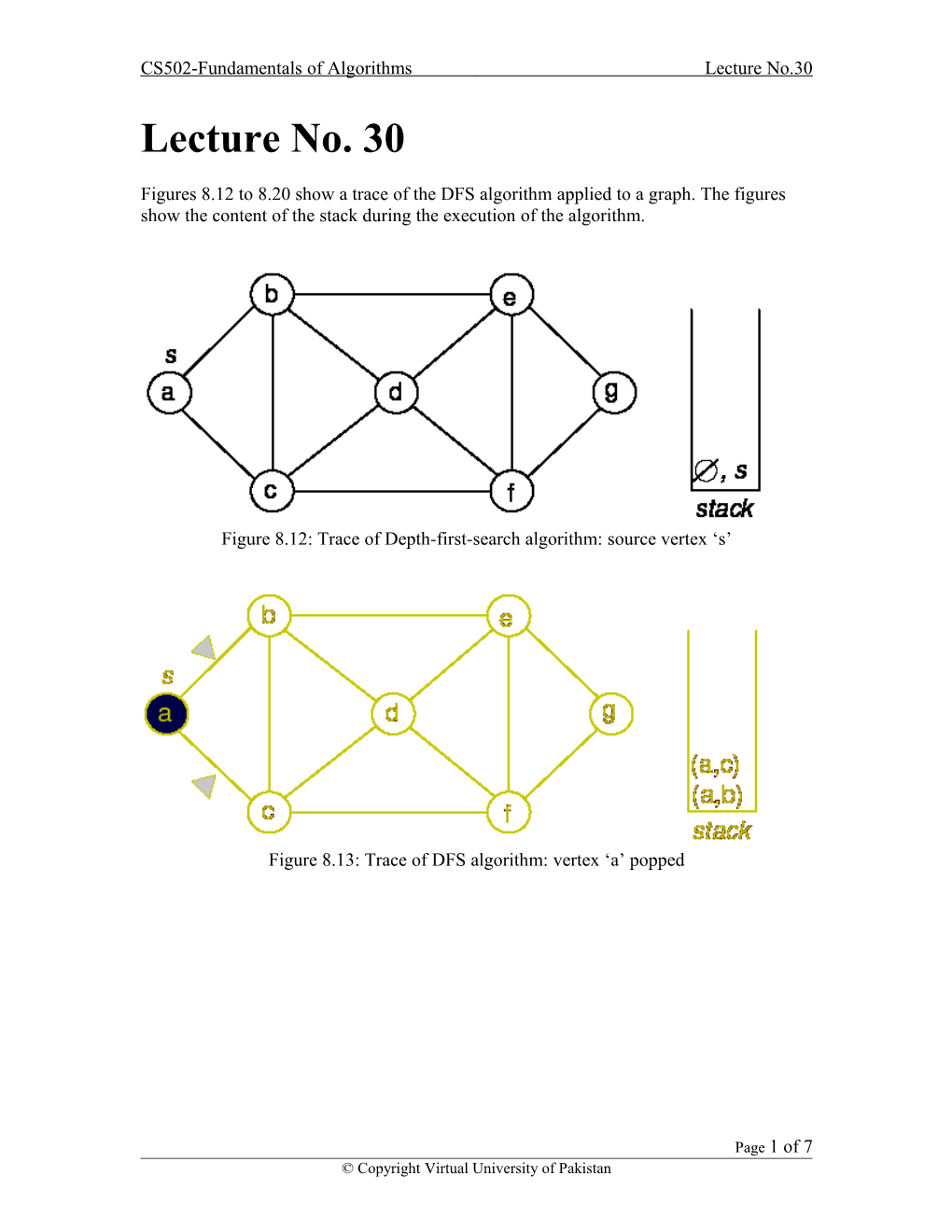# CS502-Fundamentals of Algorithmslecture No.30CS502-Fundamentals of AlgorithmsLecture No.30

Lecture No. 30

Figures 8.12 to 8.20 show a trace of the DFS algorithm applied to a graph. The figures show the content of the stack during the execution of the algorithm.Figure 8.12: Trace of Depth-first-search algorithm: source vertex ‘s’Figure 8.13: Trace of DFS algorithm: vertex ‘a’ poppedFigure 8.14: Trace of DFS algorithm: vertex ‘c’ poppedFigure 8.15: Trace of DFS algorithm: vertex ‘f’ poppedFigure 8.16: Trace of DFS algorithm: vertex ‘g’ poppedFigure 8.17: Trace of DFS algorithm: vertex ‘e’ popped

Figure 8.18: Trace of DFS algorithm: vertex ‘b’ popped

Figure 8.19: Trace of DFS algorithm: vertex‘d’ popped

Figure 8.20: Trace of DFS algorithm: the final DFS tree

Each execution of line 3 or line 8 in the TRAVERSE-DFS algorithm takes constant time. So the overall running time is O(V + E). Since the graph is connected, V E + 1, this is O(E).

If we implement the bag by using a queue, we have breadth-first search (BFS). Each execution of line 3 or line 8 still takes constant time. So overall running time is still O(E).

If the graph is represented using an adjacency matrix, the finding of all the neighbors of vertex in line 7 takes O(V) time. Thus depth-first and breadth-first take O(V2) time overall.

Either DFS or BFS yields a spanning tree of the graph. The tree visits every vertex in the graph. This fact is established by the following lemma:

Lemma:

The generic TRAVERSE(S) marks every vertex in any connected graph exactly once and the set of edges (v, parent(v)) with parent(v) form a spanning tree of the graph.

Proof:

First, it should be obvious that no vertex is marked more than once. Clearly, the algorithm marks s. Let v s be a vertex and let s  · · ·u v be a path from s to v with the minimum number of edges.

Since the graph is connected, such a path always exists. If the algorithm marks u, then it must put (u, v) into the bag, so it must take (u, v) out of the bag at which point v must be marked. Thus, by induction on the shortest-path distance from s, the algorithm marks every vertex in the graph.

Call an edge (v, parent (v)) with parent (v) , a parent edge. For any node v, the path of parent edges v parent (v) parent (parent (v)) . . . eventually leads back to s. So the set of parent edges form a connected graph.

Clearly, both end points of every parent edge are marked, and the number of edges is exactly one less than the number of vertices. Thus, the parent edges form a spanning tree.

8.1.4 DFS - Timestamp Structure

As we traverse the graph in DFS order, we will associate two numbers with each vertex. When we first discover a vertex u, store a counter in d[u]. When we are finished processing a vertex, we store a counter in f[u]. These two numbers are time stamps.

Consider the recursive version of depth-first traversal.

The DFSVISIT routine is as follows:

Page1 of 7